Author: Oscar Cronquist Article last updated on February 01, 2019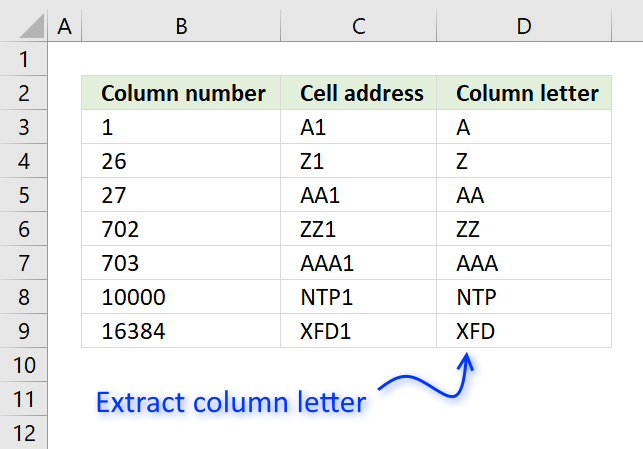Use the following formula to convert a column number to a column letter:

=LEFT(ADDRESS(1, B3, 4), MATCH(B3, {1; 27; 703}))

The formula is entered in cell D3 shown in the image above, the column number is in cell B3.

### Explaining formula in cell D3

You can follow along if you start the "Evaluate Formula" tool. You will find it on tab "Formula" on the ribbon.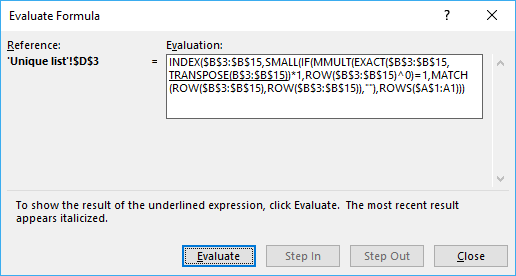Press with left mouse button on "Evaluate" button to move to the next calculation step.

#### Step 1 - Create a relative cell reference based on row and column number

The ADDRESS function returns a cell reference depending on what you use in the first (row) and second (column) argument.

The third argument lets you choose the type of cell reference the ADDRESS function returns. 4 is a relative cell reference.

becomes

and returns A1.

#### Step 2 - Remove last characters based on column number

The column letters start with A and ends with XFD. A is column 1, AA is column 27, the first column reference that contains two letters.

AAA is the first column containing 3 letters and the corresponding column number is 703.

The MATCH function returns the position in the array of the largest value that is smaller than the lookup value (B3).

MATCH(B3, {1; 27; 703})

becomes

MATCH(1, {1; 27; 703})

and returns 1. The cell reference must have a single column letter.

#### Step 3 - Extract a given number of characters from the start of the string

LEFT(ADDRESS(1, B3, 4), MATCH(B3, {1; 27; 703}))

becomes

LEFT("A1", 1)

and returns A in cell D3.

## Convert column letter to column number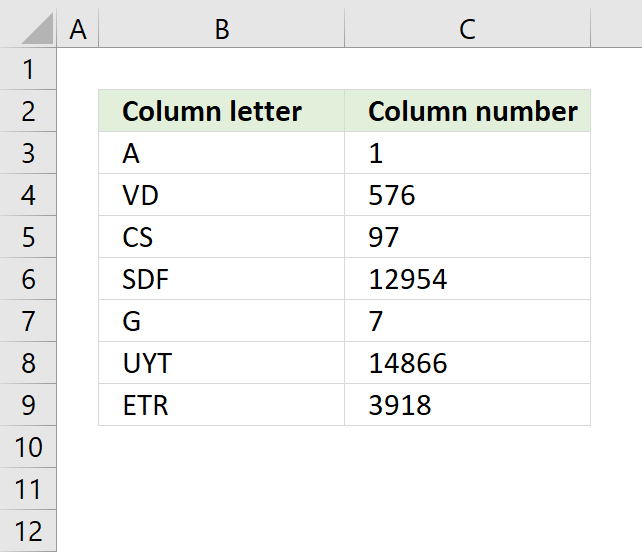The following formula converts a column letter to the corresponding column number.

Formula in cell C3:

=COLUMN(INDIRECT(B3&"1"))

If you don't want to use the INDIRECT function because it is volatile and may cause your worksheet to slow down considerably if used extensively, use this array formula.

If you rather use a regular formula, try this:

### Explaining formula in cell C3

#### Step 1 - Create a cell reference from a text string

The ampersand character & concatenates the value in cell B3 with 1.

The INDIRECT function converts the text string to a cell reference.

INDIRECT(B3&"1")

becomes

INDIRECT("A"&"1")

becomes

INDIRECT("A1")

and returns A1.

#### Step 2 - Return column from cell reference

COLUMN(INDIRECT(B3&"1"))

becomes

COLUMN(A1)

and returns 1 in cell C3.

## Convert column number to column letter (VBA)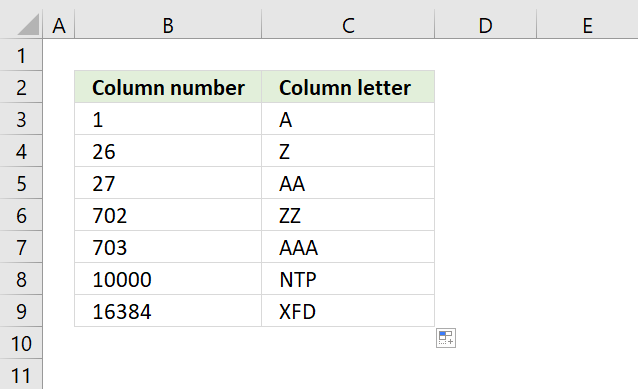User defined function in cell C3:

=ColumnLetter(B3)

#### VBA code

```Function ColumnLetter(col As Integer) As String
End Function
```

#### Where to copy the code?

1. Copy above custom function
2. Go to VBA Editor (Alt+F11)
3. Press with left mouse button on "Insert" on the top menu
4. Press with left mouse button on "Module" to insert a module to your workbook
5. Paste code into the code window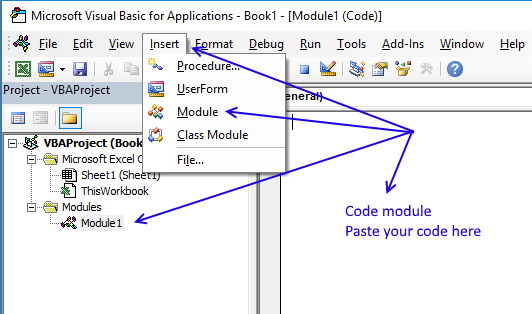To be able to use the user defined function next time you open your workbook you need to save the workbook as a macro-enabled workbook.

1. Press with left mouse button on "File" on the menu, or if you have an earlier version of Excel, press with left mouse button on the office button.
2. Press with left mouse button on "Save As"
3. Press with left mouse button on file extension drop-down list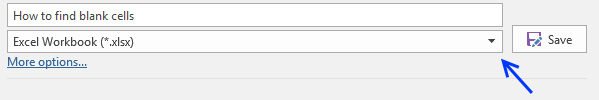4. Change the file extension to "Excel Macro-Enabled Workbook (*.xlsm)".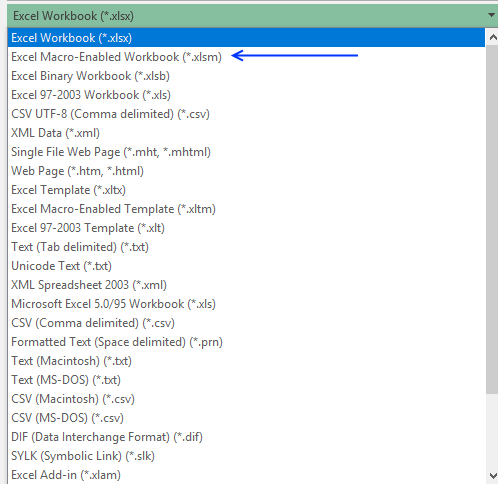## Convert column letter to column number (VBA)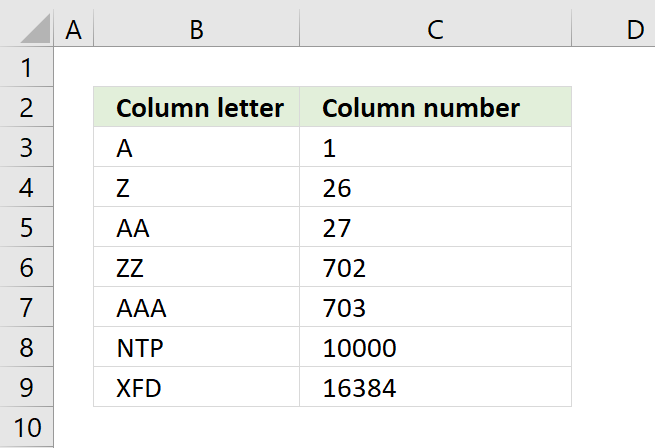User defined function in cell C3:

=ColumnNumber(B3)

#### VBA code

```Function ColumnNumber(col As String) As Long
ColumnNumber = Columns(col).Column
End Function
```